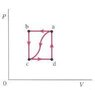# Thermodynamics- PV diagram

uchicago2012

## Homework Statement

For point A in the figure (See Figure 1), the pressure is 3 atm, the volume is .08 m3 and the internal energy is 750 J. At point C the values are 1.2 atm, .03 m3, and 420 Joules.
(a) For the cyclic process abcda, how much heat is exchanged with the environment?
(b) For the process abc, what is the change in internal energy?

## Homework Equations

delta U = Q - W
where U = internal energy, Q = heat, and W = work

## The Attempt at a Solution

for (a), I found Q = 9119.25 J because it's a cyclic process, so delta U = 0 and Wnet = the area of the square. But for (b), it's not exactly clear how I'm supposed to find the change in internal energy. I can find W by finding the area under the curve, which equals 15198.75 J, but I don't know U now and I don't know Q either. All I can get is Qda > 0, which isn't particularly helpful.

#### Attachments

•Figure1.jpg
2.5 KB · Views: 415